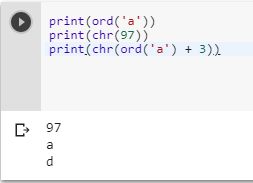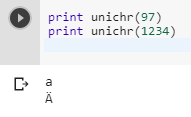2 views
in Python

How do I get the ASCII value of a character as an int in Python?

by (106k points)

To get the ASCII value of a character in Python we have a function called as ord(), it would get the int value of the char. And in case if you want to convert back char into int then you can use the function chr() which does the trick.

Below is an example that illustrates the use of ord() and char() function respectively:-

print(ord('a'))

print(chr(97))

print(chr(ord('a') + 3))If you are using Python 2, then there is also a function which Tells the ASCII value which is unichr():-

print unichr(97)

print unichr(1234)